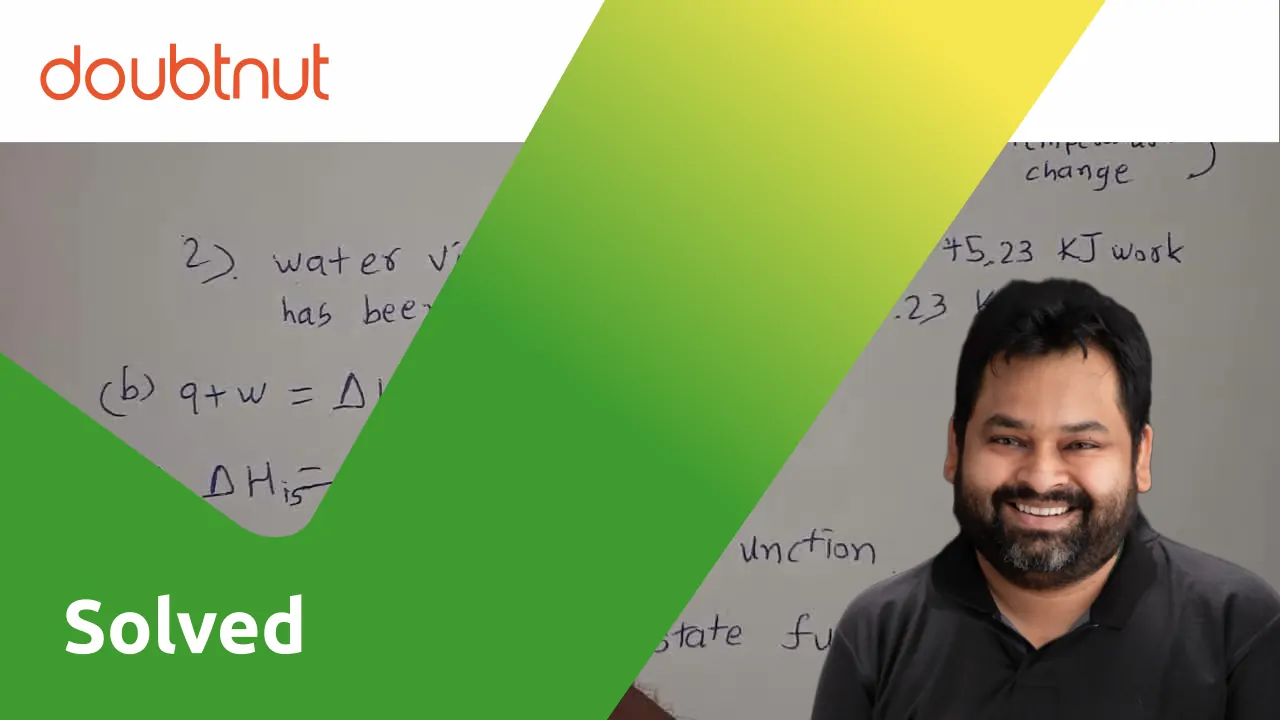Doubtnut App - Get 50 Lakh+ video solutions better than GoogleInstall Now

# Which of the following is not a state functions?Solution in Gujrati

A

(i) and (iv)

B

(ii), (iii) and (iv)

C

(i), (ii) and (iii)

D

(ii) and (iii)

Text Solution
Verified by Experts

## We know that q (heat and work (w)) are not state functions but (q + w) is a state functions. H - TS (i.e. G) is also a state function. Thus (ii) and (iii) are pot state function, so the correct answer is option (D).